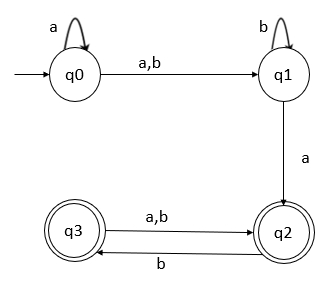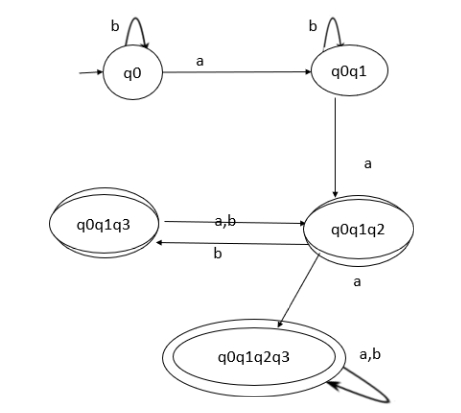# Give an example problem of converting NFA to DFA.

## Problem

Consider a Non-deterministic finite automata (NFA) and convert that NFA into equivalent Deterministic Finite Automata (DFA).## Solution

Let’s construct NFA transition table for the given diagram −

States\inputsab
->q0{q0,q1}q0
q1q2q1
q2q3q3
q3-q2

DFA cannot have multiple states. So, consider {q0,q1} as a single state while constructing DFA.

Let’s convert the above table into equivalent DFA

States\inputsab
->q1[q0,q1]q0
[q0,q1][q0q1q2][q0q1]
*[q0q1q2][q0q1q2q3][q0q1q3]
*[q0q1q2q3][q0q1q2q3][q0q1q2q3]
*[q0q1q3][q0q1q2][q0q1q2]

In DFA the final states are q2 and q3, wherever q2 and q3 are present that state becomes a final state.

Now the transition diagram for DFA is as follows −